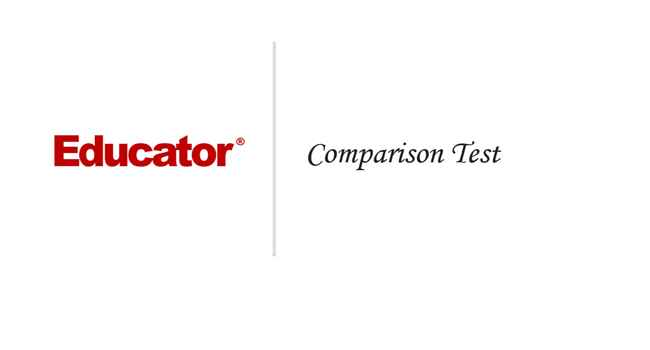Professor Murray

Comparison Test

Slide Duration:

Integration by Parts

24m 52s

Intro
0:00
Important Equation
0:07
Where It Comes From (Product Rule)
0:20
Why Use It?
0:35
Lecture Example 1
1:24
Lecture Example 2
3:30
Shortcut: Tabular Integration
7:34
Example
7:52
Lecture Example 3
10:00
Mnemonic: LIATE
14:44
Ln, Inverse, Algebra, Trigonometry, e
15:38
-1
-2
Integration of Trigonometric Functions

25m 30s

Intro
0:00
Important Equation
0:07
Powers (Odd and Even)
0:19
What To Do
1:03
Lecture Example 1
1:37
Lecture Example 2
3:12
Half-Angle Formulas
6:16
Both Powers Even
6:31
Lecture Example 3
7:06
Lecture Example 4
10:59
-1
-2
Trigonometric Substitutions

30m 9s

Intro
0:00
Important Equations
0:06
How They Work
0:35
Example
1:45
Remember: du and dx
2:50
Lecture Example 1
3:43
Lecture Example 2
10:01
Lecture Example 3
12:04
-1
-2
Partial Fractions

41m 22s

Intro
0:00
Overview
0:07
Why Use It?
0:18
Lecture Example 1
1:21
Lecture Example 2
6:52
Lecture Example 3
13:28
-1
-2
Integration Tables

20m

Intro
0:00
Using Tables
0:09
Match Exactly
0:32
Lecture Example 1
1:16
Lecture Example 2
5:28
Lecture Example 3
8:51
-1
-2
Trapezoidal Rule, Midpoint Rule, Left/Right Endpoint Rule

22m 36s

Intro
0:00
Trapezoidal Rule
0:13
Graphical Representation
0:20
How They Work
1:08
Formula
1:47
Why a Trapezoid?
2:53
Lecture Example 1
5:10
Midpoint Rule
8:23
Why Midpoints?
8:56
Formula
9:37
Lecture Example 2
11:22
Left/Right Endpoint Rule
13:54
Left Endpoint
14:08
Right Endpoint
14:39
Lecture Example 3
15:32
-1
-2
Simpson's Rule

21m 8s

Intro
0:00
Important Equation
0:03
Estimating Area
0:28
Difference from Previous Methods
0:50
General Principle
1:09
Lecture Example 1
3:49
Lecture Example 2
6:32
Lecture Example 3
9:07
-1
-2
Improper Integration

44m 18s

Intro
0:00
Horizontal and Vertical Asymptotes
0:04
Example: Horizontal
0:16
Formal Notation
0:37
Example: Vertical
1:58
Formal Notation
2:29
Lecture Example 1
5:01
Lecture Example 2
7:41
Lecture Example 3
11:32
Lecture Example 4
15:49
Formulas to Remember
18:26
Improper Integrals
18:36
Lecture Example 5
21:34
Lecture Example 6 (Hidden Discontinuities)
26:51
-1
-2
Section 2: Applications of Integrals, part 2
Arclength

23m 20s

Intro
0:00
Important Equation
0:04
Why It Works
0:49
Common Mistake
1:21
Lecture Example 1
2:14
Lecture Example 2
6:26
Lecture Example 3
10:49
-1
-2
Surface Area of Revolution

28m 53s

Intro
0:00
Important Equation
0:05
Surface Area
0:38
Relation to Arclength
1:11
Lecture Example 1
1:46
Lecture Example 2
4:29
Lecture Example 3
9:34
-1
-2
Hydrostatic Pressure

24m 37s

Intro
0:00
Important Equation
0:09
Main Idea
0:12
Different Forces
0:45
Weight Density Constant
1:10
Variables (Depth and Width)
2:21
Lecture Example 1
3:28
-1
-2
Center of Mass

25m 39s

Intro
0:00
Important Equation
0:07
Main Idea
0:25
Centroid
1:00
Area
1:28
Lecture Example 1
1:44
Lecture Example 2
6:13
Lecture Example 3
10:04
-1
-2
Section 3: Parametric Functions
Parametric Curves

22m 26s

Intro
0:00
Important Equations
0:05
Slope of Tangent Line
0:30
Arc length
1:03
Lecture Example 1
1:40
Lecture Example 2
4:23
Lecture Example 3
8:38
-1
-2
Polar Coordinates

30m 59s

Intro
0:00
Important Equations
0:05
Polar Coordinates in Calculus
0:42
Area
0:58
Arc length
1:41
Lecture Example 1
2:14
Lecture Example 2
4:12
Lecture Example 3
10:06
-1
-2
Section 4: Sequences and Series
Sequences

31m 13s

Intro
0:00
Definition and Theorem
0:05
Monotonically Increasing
0:25
Monotonically Decreasing
0:40
Monotonic
0:48
Bounded
1:00
Theorem
1:11
Lecture Example 1
1:31
Lecture Example 2
11:06
Lecture Example 3
14:03
-1
-2
Series

31m 46s

Intro
0:00
Important Definitions
0:05
Sigma Notation
0:13
Sequence of Partial Sums
0:30
Converging to a Limit
1:49
Diverging to Infinite
2:20
Geometric Series
2:40
Common Ratio
2:47
Sum of a Geometric Series
3:09
Test for Divergence
5:11
Not for Convergence
6:06
Lecture Example 1
8:32
Lecture Example 2
10:25
Lecture Example 3
16:26
-1
-2
Integral Test

23m 26s

Intro
0:00
Important Theorem and Definition
0:05
Three Conditions
0:25
Converging and Diverging
0:51
P-Series
1:11
Lecture Example 1
2:19
Lecture Example 2
5:08
Lecture Example 3
6:38
-1
-2
Comparison Test

22m 44s

Intro
0:00
Important Tests
0:01
Comparison Test
0:22
Limit Comparison Test
1:05
Lecture Example 1
1:44
Lecture Example 2
3:52
Lecture Example 3
6:01
Lecture Example 4
10:04
-1
-2
Alternating Series

25m 26s

Intro
0:00
Main Theorems
0:05
Alternation Series Test (Leibniz)
0:11
How It Works
0:26
Two Conditions
0:46
Never Use for Divergence
1:12
Estimates of Sums
1:50
Lecture Example 1
3:19
Lecture Example 2
4:46
Lecture Example 3
6:28
-1
-2
Ratio Test and Root Test

33m 27s

Intro
0:00
Theorems and Definitions
0:06
Two Common Questions
0:17
Absolutely Convergent
0:45
Conditionally Convergent
1:18
Divergent
1:51
Missing Case
2:02
Ratio Test
3:07
Root Test
4:45
Lecture Example 1
5:46
Lecture Example 2
9:23
Lecture Example 3
13:13
-1
-2
Power Series

38m 36s

Intro
0:00
Main Definitions and Pattern
0:07
What Is The Point
0:22
0:45
Interval of Convergence
2:42
Lecture Example 1
3:24
Lecture Example 2
10:55
Lecture Example 3
14:44
-1
-2
Section 5: Taylor and Maclaurin Series
Taylor Series and Maclaurin Series

30m 18s

Intro
0:00
Taylor and Maclaurin Series
0:08
Taylor Series
0:12
Maclaurin Series
0:59
Taylor Polynomial
1:20
Lecture Example 1
2:35
Lecture Example 2
6:51
Lecture Example 3
11:38
Lecture Example 4
17:29
-1
-2
Taylor Polynomial Applications

50m 50s

Intro
0:00
Main Formulas
0:06
Alternating Series Error Bound
0:28
Taylor's Remainder Theorem
1:18
Lecture Example 1
3:09
Lecture Example 2
9:08
Lecture Example 3
17:35
-1
-2
Bookmark & Share Embed

## Copy & Paste this embed code into your website’s HTML

Please ensure that your website editor is in text mode when you paste the code.
(In Wordpress, the mode button is on the top right corner.)
×
• - Allow users to view the embedded video in full-size.
Since this lesson is not free, only the preview will appear on your website.

• ## Related Books1 answer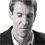Last reply by: Dr. William MurrayThu Aug 4, 2016 6:02 PMPost by Peter Ke on July 30, 2016For example 3, why can't we go straight to testing if the exponent is bigger than or less than 1 instead of wasting time doing the limit comparison test?1 answerLast reply by: Dr. William MurrayThu Apr 24, 2014 6:18 PMPost by Brandyn Albrecht on April 22, 2014Quick question. taking an/bn is just to figure out if the series are similar or whatever in terms of divergence, but for example 1 just to double check I took (1/n+1)/1/n and got n/(n+1) which converges to 1, which is a positive real number, which means an and bn should act similarly right? But they don't because an is smaller than bn, so does the an/bn approach only work for more confusing questions like example 3, and not for all questions in general?3 answersLast reply by: Dr. William MurrayTue Dec 17, 2013 9:09 PMPost by Xenia Jeanty on November 30, 2013lecture example 3 why is sqrt n/n same as 1/sqrt n? Can you please explain how did we get there?1 answerLast reply by: Dr. William MurrayTue Aug 13, 2013 5:24 PMPost by charles daniel on July 24, 2013lecture example 3 in the denominator n/n^2 should be equal to zero ..right?..why is it equal to one1 answerLast reply by: Dr. William MurrayTue Aug 13, 2013 5:21 PMPost by charles daniel on July 24, 2013lecture example 3 .. how is the bn of root(2n+17)/n become root n/n1 answerLast reply by: Dr. William MurrayTue Apr 16, 2013 8:27 PMPost by Alena Schwartsman on April 14, 2013Thank you Dr. Murray, concise and to the point. I like how you repeat the test's requirements with every example; easier to memorize the tests this way. Overall, I love how you explain things! Thank you again!1 answerLast reply by: Dr. William MurrayWed Apr 3, 2013 6:43 PMPost by Rohail Tariq on April 1, 2013cool1 answerLast reply by: Dr. William MurrayWed Apr 3, 2013 6:42 PMPost by Ahmad Alshammari on September 13, 2011why you put .. 3^(n-2) !!1 answerLast reply by: Dr. William MurrayWed Apr 3, 2013 6:34 PMPost by Srinivasa Rao RAVELLA on April 14, 2011how would you should tan(1/x) is convergent with the limit comparison test1 answerLast reply by: Dr. William MurrayWed Apr 3, 2013 6:26 PMPost by Romin Abdolahzadi on April 4, 2011Regarding the limit comparison test: If the limit is equal to 0, then if Bn converges then An also converges!

### Comparison Test

Main theorems:

Suppose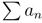and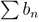are series with positive terms. (is given to you, and you createyourself.)

Comparison Test:

• If an <bn for all n andconverges, thenconverges.

• If an >bn for all n anddiverges, thendiverges.

Limit Comparison Test: Suppose lim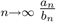= some finite, positive (not 0) number.

• Then ifconverges thenconverges, and

• ifdiverges thendiverges.

Hints and tips:

• Like the Integral Test, the Comparison Tests only work for series with positive terms. However, after you learn about absolute convergence later, you may be able to use them for series with some negative terms by taking their absolute value and seeing if they are absolutely convergent.

• The idea with these tests is that you are given a series. You come up with the seriesyourself. It should be similar in form to, but simpler to analyze.

• Often you will taketo be a p-series or geometric series. Remember the differences between these two and the conditions under which each one converges or diverges.

• The Comparison Tests are two-way tests − you can use them to conclude that a series converges or that it diverges. However, it’s very important that the inequalities go the right way. If you have a series that is bigger than a known convergent series, the Comparison Test tells you nothing.

• And if you have a series that is smaller than a known divergent series, the Comparison Test tells you nothing.

• Because the inequalities must go the right way, it’s often useful to get some intuition about whether a series converges or diverges before setting up a comparison. It’s useful to remember the ranking of functions: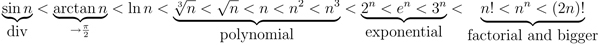• When you have a complicated rational expression, focus on the biggest term in the numerator and the biggest term in the denominator.

### Comparison Test

Does the series ∑ [1/(2 + n4)] converge?
• Determine an appropriate un for the Comparison Test
• Consider un = [1/(n4)]
• Consider n = 2, then an ≤ un
[1/(n4)] p = 4 > 1, thus the series un converges.
Since un converges, then an converges
Does the series ∑ [1/(n2 − 50)] converge?
• Determine an appropriate un for the Comparison Test
• Consider un = [1/(n2)]
• Consider n = 3, then an ≤ un
[1/(n2)] p = 2 > 1, thus the series un converges
Since un converges, then an converges
Does the series ∑ [1/(√{n + 6})] converge?
• Determine an appropriate un for the Comparison Test
• Consider un = [1/(n1/2)]
• Consider n = 3, then anun
• Determine ∑ un diverges with P - series properties
• [1/(n1/2)]
• p = [1/2] ≤ 1, thus the series un diverges
Since un diverges, then an diverges
Does the series 1 + [1/2] + [1/(√7 )] + ... + [1/(√{3n − 2} )] + ... converge?
• Represent the series as a summation
• 1 + [1/2] + [1/(√7 )] + ... + [1/(√{3n − 2} )] + ... = ∑ [1/(√{3n − 2} )]
• Determine an appropriate un for the Comparison Test
• Consider un = [1/(√{3n} )] = [1/(√3 )]( [1/(√n )] )
• Consider n = 2, then anun
[1/(√{3n} )] = ∑ [1/(√3 )]( [1/(√n )] ) = [1/(√3 )]∑ [1/(√n )] p = [1/2] ≤ 1, thus the series un diverges
Since un diverges, then an diverges
If ∑ un diverges and an ≤ un, does ∑ an diverge?
Analyze the conditions for the Comparison Test
No, because the condition requires anun
Does the series ∑ [(n + 1)/(n2 + 2)] converge?
• Determine an appropriate un for the Comparison Test
• Consider un = [1/(n)]
• Consider n = 3, then anun
[1/(n)] Since it's a Harmonic Series, the series un diverges
Since un diverges, then an diverges
Does the series ∑ [1/(√{n3 + 6} )] converge?
• Determine an appropriate un for the Comparison Test
• Consider un = [1/(√{n3} )]
• Consider n = 3, then an ≤ un
• Determine ∑ un converges with P − series properties
• [1/(√{n3} )] = ∑ [1/(n3/2)]
• p = [3/2] > 1, thus the series un converges
Since un converges, then an converges
If ∑ un converges and anun, does ∑ an converge?
Analyze the conditions for the Comparison Test
No, because the condition requires an ≤ un
Does the series ∑ [(n3)/((n + 1)2)] converge?
• Determine an appropriate un for the Comparison Test
• Consider un = n
• Consider n = 3, then an ≤ un
n = ∞ Thus the series un diverges
Since un diverges, then an diverges
Does the series ∑ [1/(5n3 + n2 + 1)] converge?
• Determine an appropriate un for the Comparison Test
• Consider un = [1/(n3)]
• Consider n = 2, then an ≤ un
• Determine ∑ un converges with P - series properties
• [1/(n3)]
• p = 3 > 1, thus the series un converges
Since un converges, then an converges
Does the series ∑[1/(7n − 3)] converge?
• Use bn = [1/n] for Limit Comparison Test
= [n/7n] = [1/7] Thus the series diverges because bn diverges
Does the series ∑[3/(19 − n)] converge?
• Use bn = [1/n] for Limit Comparison Test
= − [3n/n] = − 3 Thus the series diverges because bn diverges
Does the series ∑[1/(√{n + 1} )] converge?
• Use bn = [1/(√n )] for Limit Comparison Test
= [(√n )/(√n )] = 1 Thus the series converges because bn converges
Does the series ∑[1/(n2 + 2n + 1)] converge?
• Use bn = [1/(n2)] for Limit Comparison Test
• limn → ∞ [([1/(n2 + 2n + 1)] )/([1/(n2)])] = limn → ∞ [(n2)/(n2 + 2n + 1)]
• = [(n2)/(n2)]
• = 1
Thus the series converges because bn converges
Is it appropriate to use the Limit Comparison Test on an = n3 + 3n if bn = n2?
Analyze conditions for Limit Comparison Test
No it is not appropriate because limn → ∞ [(an)/(bn)] is infinite
Does the series ∑[(n − 2)/(n3 − 1)] converge?
• Use bn = [1/(n2)] for Limit Comparison Test
• limn → ∞ [([(n − 2)/(n3 − 1)])/([1/(n2)])] = limn → ∞ [(n2( n − 2 ))/(n3 − 1)]
= [(n3)/(n3)] = 1 Thus the series converges because bn converges
Does the series ∑[(√n )/((2n − 1)2)] diverge?
• Use bn = [1/(n3/2)] for Limit Comparison Test
• limn → ∞ [([(√n )/((2n − 1)2)])/([1/(n3/2)])] = limn → ∞ [(√n (n3/2))/((2n − 1)2)]
= [(n2)/(4n2)] = [1/4] Thus the series converges because bn converges
Does the series ∑[(7n2)/(9n3 + 8n2 + 2n + 3)] diverge?
• Use bn = [1/n] for Limit Comparison Test
• limn → ∞ [([(7n2)/(9n3 + 8n2 + 2n + 3)])/([1/n])] = limn → ∞ [(7n3)/(9n3 + 8n2 + 2n + 3)]
• = [(7n3)/(9n3)]
• = [7/9]
Thus the series diverges because bn diverges
Does the series ∑[(3n2 + n)/(5n3 − 12n2 − n + 17)] diverge?
• Use bn = [1/n] for Limit Comparison Test
= [(3n3)/(5n3)] = [3/5] Thus the series diverges because bn diverges
Does the series 3 + [3/4] + [1/3] + [3/16] + ... converge?
• Analyze the series
• 3 + [3/4] + [1/3] + [3/16] + ... = ∑[3/(n2)]
• Use bn = [1/(n2)] for Limit Comparison Test
• limn → ∞ [([3/(n2)])/([1/(n2)])] = limn → ∞ [(3n2)/(n2)]
• = 3
Thus the series converges because bn converges

*These practice questions are only helpful when you work on them offline on a piece of paper and then use the solution steps function to check your answer.

### Comparison Test

Lecture Slides are screen-captured images of important points in the lecture. Students can download and print out these lecture slide images to do practice problems as well as take notes while watching the lecture.

• Intro 0:00
• Important Tests 0:01
• Comparison Test
• Limit Comparison Test
• Lecture Example 1 1:44
• Lecture Example 2 3:52
• Lecture Example 3 6:01
• Lecture Example 4 10:04

### Transcription: Comparison Test

Hi, we are checking out some more examples of the comparison test and the limit comparison test.0000

What we have right here is the sum of en/n!.0005

That one is a little tricky.0011

I want to write that one as en/n×1×2×3...×n.0013

What I notice here is this 3, 4, up to n, all of those numbers are bigger than or equal to 3.0031

You will see why the 3 is significant as a cut off in a second.0039

This is < or = en/1×2×3, and there were n-2 factors there.0044

So 3n-2.0062

Just to make the algebra a little bit cleaner, I am going to multiply the top and bottom by 32.0064

32 × en/1 × 2, now I can say that is 3n.0071

I just multiplied in 32 × the top and bottom.0078

So that I can have an n in the exponent instead of an n-2.0082

This in turn is, 32 is 9/2 × en/3n.0085

This is (e/3)n.0094

That is the series that I am going to use as my bn.0100

Remember an is always the series that you are given.0102

The point here is that an is < or = to bn.0109

The sum of bn converges.0114

Why does it converge?0118

Because, bn is just a constant 9/2 × e/3n.0121

When you have a constant raised to the n power, it is a geometric series.0128

So you look at the common ratio, well here r = e/3.0136

I do not know the exact value of e, but I know it is about 2.7.0149

The key thing there is that 2.7/3 is less than 1.0154

Remember 1 is the cutoff to check when a geometric series converges.0159

We have that e/3 < 1, so our common ratio < 1.0165

That means the sum of bn converges.0171

So, our smaller series an, must also converge by the comparison test0175

Not the limit comparison, the original comparison test.0185

So, now it should be evident why I cut things off between 2 and 3 here.0193

I was kind of looking ahead to this common ratio.0202

I knew that e is about 2.7.0206

So, I saw that I had a bunch of numbers bigger than that in the denominator,0211

If I cut it off between 2 and 3.0218

That is why I cut off all of these number bigger than 3.0221

I used those to build the geometric series,0225

And then I just had to save the 1 and 2 separately.0229

1 × 2 just gave me a two in the denominator and did not really affect things later on.0232

The important thing was to save the e/3, and compare it with a geometric series.0238

Since we know the geometric series converges, and we have something smaller,0242

We can say our own series converges by the comparison test.0250

The last example that I want to do here is a little more complicated.0000

We have the series of n4+3n-8/3n7+6n5.0006

You see something complicated like this and it is important to focus on which terms are really going to affect it,0014

And which terms are not really going to play much role.0020

The key thing here is the two biggest terms, n4, in the numerator, and n7 in the denominator.0024

It looks like if you strip away the extraneous stuff there,0030

It looks like the sum of n4/n7.0038

That in turn would simplify down to 1/n3.0041

OK, I am going to call my 1/n3, that is going to be the bn that I create.0051

This entire given series, that is an.0057

We will look at an vs. bn,0062

We will divide them together and try to go for something using the limit comparison test.0065

an/bn is n4+3n-8/3n7+6n5.0069

bn is 1/n3,0081

But we can flip that up into the numerator so we can write that as n3/1.0084

That turns into n7+3n4-8n3/3n7+6n5.0094

Now you look at something like this and identify the biggest term anywhere,0107

Which is an n7 in the top and the bottom and you divide by it.0113

So, you get 1+3/n3-8/n4/3+6/n2.0116

All of these other terms, go to 0.0134

This whole thing goes to 1/3.0140

The key thing about the 1/3 there, is only that it is a finite number, it is not infinity and it is not 0.0144

If you get a finite number there, the limit comparison test says that your given series does whatever the other series does.0152

It does the same thing that the other series does.0160

In this case, since the series we made ourselves, the bn,0164

Well that is 1/n3, that is a p series and p is 3.0171

That converges.0176

The important thing about 3 there is that it is > 1.0187

Anything > 1 makes that converge.0190

Since that converges, we can say that the sum/an also converges.0192

Our justification there is the limit comparison test.0203

Either both series converge, or both series diverge.0208

Since the one we introduced converged, the given one converges as well.0211

To recap there, we are given some complicated series here.0217

We identify the important elements and use those important elements to build our own series that we introduce.0222

We then divide them together, take the limit,0230

If we get a finite non-0 limit,0234

Then we can say that both series do the same thing.0237

Hopefully the series that we introduced is a simple enough one where we can look at it and quickly say if it diverges or converges.0240

In this case it is a p series, and p is bigger than 1, so it converges.0248

So, we can say that the given series converges as well.0254

Thanks for watching, this is educator.com0259

Hi, this is Will Murray and we are here today to talk about the comparison test.0000

The way this works is that you will be given a series that we are going to call an,0006

And you create your own series that we will call bn.0015

Then you will try to compare these 2 series to each other.0020

The way this works is, the one you create, if that one converges,0024

And the one that you are given is smaller than it,0029

Then you can say that the given series converges as well.0032

On the other hand, if the one you create diverges,0037

And the given series is bigger than the one you created,0040

Then you can say the given series diverges.0045

Now it is very important to get these inequalities going the right way.0048

If these inequalities are going the wrong way, then the comparison test does not tell you anything.0053

We will see some examples that illustrate the difference between those inequalities going the right way and going the wrong way.0057

We will be using a second test called the limit comparison test.0067

It starts out the same way,0069

You will be given a series, then you create your own series,0071

But then instead of checking the inequalities,0074

What you do is you divide those 2 series together and then take the limit as n goes to infinity.0077

If you get a finite and positive number, it cannot be 0, it has to be a positive number,0084

Then you can say whatever the series you created does, converges or diverges,0091

You can say that the given series does the same thing.0097

let us check that out with some examples.0101

The first one here is the series of 1/n+1.0105

That is the given series, the an.0109

When you look at this series, the simplest series that this seems to resemble,0113

Is the series 1/n.0123

Because 1/n+1, is about the same as 1/n.0126

So that is the series we create ourselves and we call the bn.0131

Now let us try comparing those to each other.0134

1/n+1 works as 1/n, well n+1 has a bigger denominator.0138

Which means that 1/n is bigger.0146

So, 1/n is bigger, the bn.0149

So, the an is less than the bn.0155

The sum of bn, that is 1/n, diverges.0162

We know that we saw that one before and that was the harmonic series.0170

Or, you can think of it as the p series, with p = 1.0178

We know that series diverges.0182

What we have is a series that is less than it.0185

If a series is less than a divergent series, that is not going the right way to use the comparison test.0189

So, comparison fails to give us an answer here.0197

Tell us nothing.0203

So, the comparison test does not tell us what this series does.0207

Instead, you have to try another test,0212

For example, you could try the integral test and that actually will give you a good answer.0215

You can check that out with the integral test.0223

It turns out that this series diverges,0226

But the important thing is that you cannot use the comparison test on this one.0228

Let us try it out with another one.0230

3n/2n - 1,0233

That looks like the sum of 3n/2n.0240

So an is the given series, for our bn, we will use the sum of 3n/2n.0249

Then we will compare those to each other.0255

So, 3n/2n-1 vs. 3n/2n.0260

Well 2n-1 is a smaller denominator which means that 3n/2n-1 is actually a bigger number.0268

What that is saying is that an is bigger than bn.0278

We know that the sum of the bn's diverges.0285

The reason we know that is because it is a geometric series.0295

The common ratio is 3/2, because 3/n/2n is just 3/2n, and 3/2 is bigger than 1.0300

So that is a geometric series that diverges,0309

And here we have a bigger series, so this bigger series, we can say it diverges by the comparison test.0313

In this case, the inequality did go the right way.0329

So, we get a conclusion by the comparison test.0333

Just a recap there, we looked at the series we were given, we tried to find the series that was similar to it,0338

And simple enough that we could answer pretty quickly whether it converged or diverged.0346

In this case it was a geometric series.0350

Then we compared them to each other.0354

The inequality does go the right way, so we can make this conclusion.0356

Next example I wanted to try is the sum of sqrt(2n+17)/n.0361

Now, this one most closely looks like,0369

Well, the sqrt(2n+17) that is really more or less the sqrt(n).0376

sqrt(n)/n, and so that in turn is sqrt(n)/n 1/sqrt(n).0385

That is the series we are going to use as our bn.0398

This series is our an.0401

What we are going to do is divide those together because we are going to try to use the limit comparison test this time.0404

So we look at aN/bn,0411

And that is the sqrt(2n+17)/n.0414

All of that divided by bn, which is 1/sqrt(n).0420

We can flip that fraction in the denominator up the numerator,0424

So we get sqrt(2n+17).0429

The sqrt(2n+17) × sqrt(n)/n,0435

Which is sqrt(2n2) + 17n/n.0444

If we look at the top and bottom there, the biggest terms we have in the top, we have an n2 with a square root over it.0451

That is essentially n and in the bottom we have n.0457

So we would divide top and bottom by n, and we get,0463

If we bring that top end under the square root, we get 2+17/n.0467

Because when the n comes under the square root it turns into an n2.0472

Then just 1 in the denominator.0477

17/n goes to 0.0480

So, the whole thing goes to sqrt(2).0483

The important thing about the sqrt(2) is that it is a finite number, it is not infinity.0488

It is not 0, so the limit comparison test applies.0493

Since the sum of bn diverges, well how do we know that,0498

That is because we can think of 1/sqrt(n) as 1/n1/2.0509

That is a p series, with p equal to 1/2.0515

The key thing there is that 1/2 < or = 1.0522

That means that bn is a divergent series.0527

The limit comparison test says that the given series an does the same thing that your series bn does.0530

So, we can conclude that the sum of an also diverges by the limit comparison test.0539

Again, the key point there is that we look at the series that we are given, and we try to find,0550

So that is the given series,0562

We try to find the given series that behaves like the series that we are given but is simpler to deal with.0565

What I did here was essentially strip away the 2 and the 17,0569

Because those were not so important, and then I called the new series bn.0574

Then we try to compare those series to each other.0578

This time by dividing.0582

If we get this finite non-zero number at the end of it,0584

That says that both series behave the same way.0588

Since we know the bn diverges, because it is a p series,0593

Then we can say the an diverges as well and justify that conclusion using the limit comparison test.0597

So, another example I would like to look at is the sum of sin(1/n).0605

The way we might think about that is,0610

let us think about the graph of sin(x).0614

As n goes to infinity, 1/n goes to 0.0618

So let us look at the graph of sin(x) when x is very near 0.0625

What we see is that the graph of sin(x),0629

This is sin(x), is very close to the graph of x as x approaches 0.0637

Sin(x) is almost the same as x.0647

Sin(1/n) the an might behave like the series bn = 1/n.0650

Because sin(x) behaves like x.0660

Let us try that out.0662

We will take the series bn = 1/n, and then we will look at an/bn.0665

Is sin(1/n)/1/n.0673

Remember as n goes to infinity, 1/n goes to 0.0679

So this is sin(0) which goes to 0.0684

1/n also goes to 0, so we have a 0/0 situation.0686

That is a situation where you can use l'Hopital's rule.0690

So l'Hopital's rule says you can take the derivatives of the top and bottom,0695

I will take the derivative of the bottom first because it is easier.0703

The derivative of 1/n is just 1/-n2.0707

The derivative of sin(1/n) is cos(1/n).0710

× derivative of 1/n by the chain rule which is -1/n2.0718

Those -1/n2's cancel.0724

We get cos(1/n) and if we take the limit of that,0727

As n goes to infinity, that is cos(0) which is 1.0735

The key thing about 1 here is only that it is a finite non-zero number.0742

If it is a finite non-zero number, that says whatever one series does, the other series does.0752

The sum of bn does what?0763

Well bn is just 1/n, and we know that is the harmonic series.0765

And it is also a p series.0771

So, either one of those is just vacationed since we already showed that it diverges.0775

It is a p series with p = 1.0780

So that is a divergent series.0788

Since that series diverges,0792

We can say that the given series an also diverges by the limit comparison test.0795

So that one was a little bit trickier, probably was not so obvious what series we should compare it too.0810

The key thing there was realizing that sin(1/n) is a lot like 1/n when n goes to infinity.0816

Because 1/n was going to 0.0825

sin(x) was very similar to x.0828

Once we figure out which series we want to compare it to, we divide them together, take the limit,0832

Which uses l'Hopital's rule, we get a finite non-zero number,0838

And that says the two series do the same thing.0843

Since one of them diverges, we can say that the given series diverges as well.0847

OR

### Start Learning Now

Our free lessons will get you started (Adobe Flash® required).